Algebraic expressions are mathematical expressions that consists of numbers, variables and mathematical operators.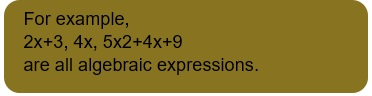# Terms, factors and coefficients

• Term: A term can be a number, a variable, or a constant multiplied by a variable or variables. Each term in an algebraic expression is separated by a positive sign or negative sign.

• Factors: If algebraic expressions are expressed as the product of numbers, variables or expressions, then each of these numbers and expressions is called the factor of algebraic expressions.

• Coefficients: When a term of an algebraic expression is made up of a constant multiplied by a variable or variables, then that constant is called a coefficient of the algebraic expression.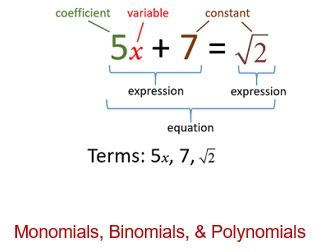# Monomials, Binomials, & Polynomials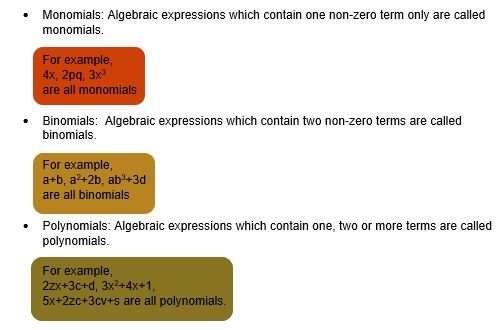# Like & Unlike terms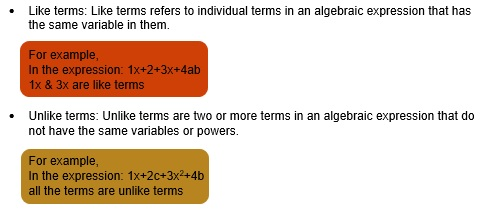# Addition & Subtraction of Algebraic Expressions

Step1: Suppose we have to add 3 expression 1a+2b+3c, 4a+5b+6c, and 7a+8b+9c
Step2: write the expression in a horizontal line and then add all the like terms: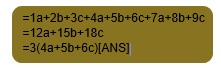Write the 3 expressions in 3 rows with like terms below each other and then add the like terms column-wise.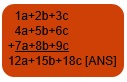• Subtraction of algebraic expression:
Step1: Suppose we have to subtract 10a+23b+45c from 67a+89b+20c

Step2: Write the above expressions in two rows such that the like terms occur one below the other. Keep the expression to be subtracted in the second row.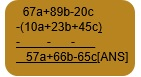# Multiplication of algebraic expressions

• In multiplication of algebraic expressions:
(i) The product of two factors having like signs is positive, and the product of two factors having unlike signs is negative.
(ii) if a is a variable and p, q are positive integers, then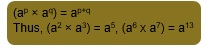• Multiplication of Two Monomials:
Product of two monomials = (product of their numerical coefficients) × (product of their variable parts)

Example 1: 1ab and -2a²b³

SOLUTION: (1ab) × (-2a²b³)

= {1 × (-2)} × {ab × a²b³}

= -2 x a1+2 x b1+3

= -2a³b⁴.

• Multiplying a polynomial by a monomial:

Multiply each term of the polynomial by the monomial, using the distributive property p × (q + r) = p × q + p × r.

EXAMPLE 2: 1x²y² × (2x² - 3xy + 4y²)

SOLUTION: 1x²y² × (2x² - 3xy + 4y²)

= (1x²y²) × (2x²) + (1x²y²) × (-3xy) + (1x²y²) × (4y²)

= 2x⁴y² - 3x³y³ + 4x²y⁴

• Multiplying a polynomial by a polynomial:

Suppose (x + y) and (p + q) are two polynomials. By using the distributive property of multiplication over addition twice, we may find their product as given below.

EXAMPLE 3: Multiply (1a³ – 2a² – a + 3) by (4 – 5a + 6a2)

SOLUTION: Arranging the terms of the given polynomials in descending power of a and then multiplying:

1a³ – 2a² – a + 3

× (4 – 5a + 6a²)
_________________________________
6a⁵ - 12a⁴ – 6a³ + 18a² ⇐ multiplication by 6a2.

- 5a⁴ + 10a³ + 5a² – 15a ⇐ multiplication by -5a.

+ 4a³ – 8a² - 4a + 12 ⇐ multiplication by 4.
_________________________________
6a⁵ – 17a⁴ + 8a³ + 15a² – 19a + 12 ⇐ multiplication by (4 – 5a + 6a²)
_________________________________

# Identities and its applications

• If an equation is true for all values of the variables in it, then that equation is called an identity.
• Some standard identities are: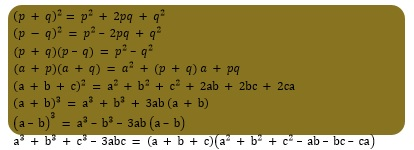EXAMPLE 1: Expand (a – 2b)3 using standard algebraic identities.

SOLUTION: (a– 2b)3 is of the form (a-b)3 where a = a and b = 2b. So we have,

(a – 2b)3 = (a)3 – (2b)3– 3(a)(2b)(a – 2b) = a3 – 8b3 – 6a2b + 12ab2 [ANS]

EXAMPLE 2: Find . Given = 12 and ab=3.

SOLUTION:

# Practice these questions

Q1) Multiply the following:
a) 11x2y and 3 b)12 and 33 c)4 and 6

Q2) Find the value of when

Q3) Simplify the following:
a) (x+2y)(3x+4y) – (5x+6y)(7x+8y) b)(9a+10b)(2x+3y) – (4a+5b)(6c+7d)

Q4) Find the product of the following using identities:
a)(8x+9y)(8x-9y) b)(10x+11y+23z)2 c)(45a-6b)3 d)(7a+8b)3

Q5) Simplify using identities:
a)121 x 121 – 10012 b)9992 c)1012 d)

Q6) If , find the value of .

Q7) Find the value of x if 12x=

Q8) Tick the pair of like terms: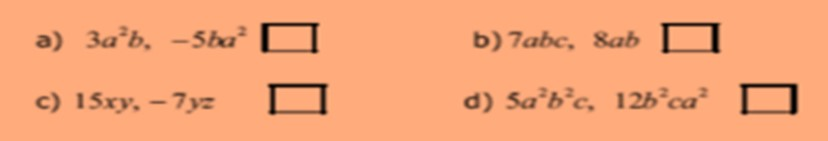Q9) If , find the value of .

# Recap

• Algebraic expressions are mathematical expressions that consists of numbers, variables and mathematical operators.
• A term can be a number, a variable, or a constant multiplied by a variable or variables. Each term in an algebraic expression is separated by a positive sign or negative sign.
• If algebraic expressions are expressed as the product of numbers, variables or expressions, then each of these numbers and expressions is called the factor of algebraic expressions.
• When a term of an algebraic expression is made up of a constant multiplied by a variable or variables, then that constant is called a coefficient of the algebraic expression.
• Algebraic expressions which contain one non-zero term only are called monomials.

Algebraic expressions which contain two non-zero terms are called binomials.